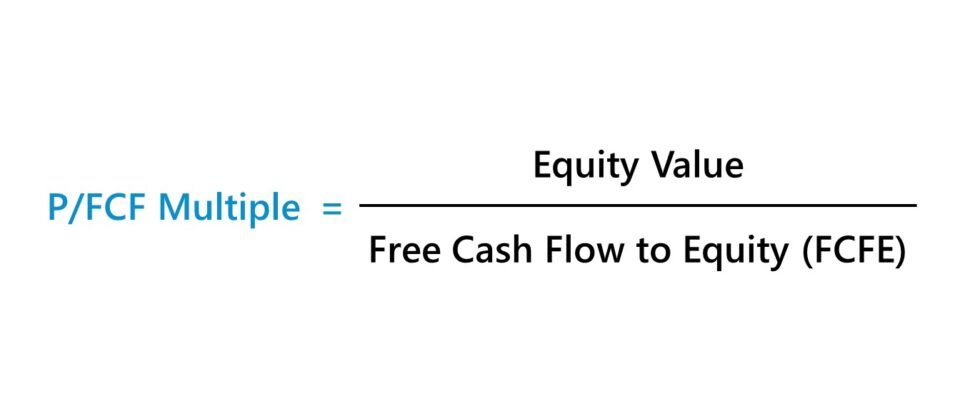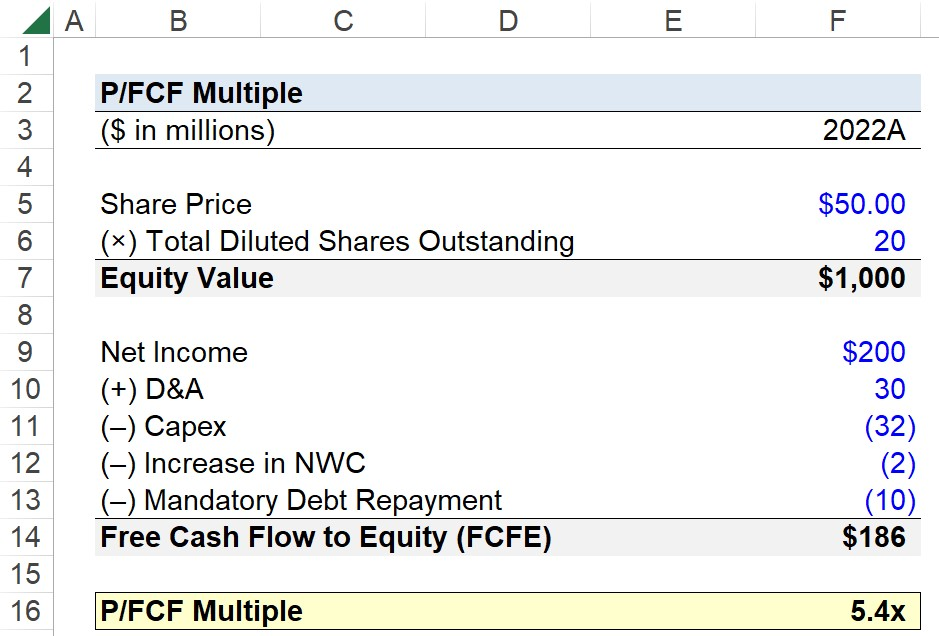Welcome to Wall Street Prep! Use code at checkout for 15% off.# P/FCF Multiple

Guide to Understanding P/FCF (Price to Free Cash Flow)## How to Calculate P/FCF?

The P/FCF multiple, or “price to free cash flow”, is the ratio between a company’s equity value and free cash flow.

• Equity Value: The equity value, also known as “market cap”, represents how much a company is worth from the perspective of only one capital provider group, the common shareholders.
• Free Cash Flow (FCF): Because the numerator is a levered metric – i.e. at its simplest, the equity value is equal to enterprise value minus net debt – the free cash flow to equity (FCFE) is the corresponding cash flow metric. Often referred to as “levered free cash flow”, FCFE is the cash flows generated by a company that belong to only common shareholders.

In order for a valuation multiple to be practical, the stakeholder(s) represented must match between the numerator and denominator; otherwise, there is an inconsistency in the ratio.

The equity value of a company is equal to the product of a company’s share price and its total number of diluted shares outstanding, i.e. debt in the capital structure is not part of the calculation.

On that note, the free cash flow to equity (FCFE) is the right metric to use alongside equity value, since FCFE is also attributable to solely common shareholders.

Unlike the free cash flow to firm (FCFF) – the cash flows applicable to all stakeholders in a company’s capital structure – FCFE is calculated after adjusting for non-equity payments such as mandatory debt amortization and interest expense (and the residual cash flows then belong to the equity holders).

## P/FCF Formula

The formula to calculate the P/FCF multiple is as follows.

P/FCF = Equity Value ÷ Free Cash Flow to Equity (FCFE)

The numerator, equity value, is calculated by multiplying the latest closing share price by the total diluted share count.

Equity Value = Market Share Price × Total Number of Diluted Shares Outstanding

As for the denominator, free cash flow to equity (FCFE), the calculation starts with net income – a post-interest profit metric – which is then adjusted for non-cash items (D&A), change in net working capital (NWC), capital expenditure (Capex), and debt repayments.

Free Cash Flow to Equity (FCFE) = Net Income + D&A  Change in NWC  Capex  Mandatory Debt Repayment

Note: If the company raised more debt capital, the proceeds are a net addition to FCFE, since the newly obtained cash can be used to issue shareholders dividends or repurchase shares.

## How to Interpret Price to Free Cash Flow Ratio?

The P/FCF ratio answers the question, “For each dollar of a company’s levered free cash flow (FCFE), how much are investors in the market currently willing to pay?”

Therefore, the higher the P/FCF multiple, the more of a premium at which the market values the company on a FCFE-basis (and vice versa for a lower P/FCF multiple).

There are two downsides to using the P/FCF multiple:

1. Fluctuations in FCFE: The free cash flow to equity (FCFE) of a company fluctuates across different periods. For instance, FCFE can be far lower if the principal on a debt obligation was repaid. In addition, the outflow of cash from capital expenditures (Capex) occurs periodically, as opposed to on a consistent basis, and a company’s working capital levels can vary based on seasonal and cyclical patterns.
2. Levered Multiple: The calculation of FCFE begins with net income, which can be distorted by discretionary decisions of management, such as the capital structure decision (i.e. reliance on debt or equity financing) and taxes. Hence, unlevered valuation multiples tend to be used far more frequently in practice, since only the core operating activities are reflected (e.g. EV/EBITDA, EV/EBIT). In contrast, levered multiples (e.g. P/E ratio, P/B ratio) can be skewed by the shortcomings of accrual accounting.
3. Lack of Standardization: Opinions can differ on the correct calculation of FCFE, especially in terms of the adjustments used to “normalize” the non-GAAP metric. In effect, the use-case of the P/FCF multiple is negatively affected by its GAAP components like net income (e.g. post-interest, interest tax shield, tax jurisdiction, non-operating items), as well as these non-GAAP adjustments.

## What’s the Difference Between P/FCF vs. Levered FCF Yield?

The EV/FCF multiple is the inverse of the levered FCF yield metric.

The levered FCF yield metric, expressed as a percentage, represents the remaining cash flows that can benefit equity shareholders, after any debt payments.

Levered FCF Yield (%) = Free Cash Flow to Equity (FCFE) ÷ Equity Value

## P/FCF Calculator – Excel Template

We’ll now move on to a modeling exercise, which you can access by filling out the form below.Submitting...

## Step 1. Equity Value Calculation (Market Capitalization)

Suppose you’re tasked with calculating the P/FCF multiple of a company using the following assumptions.

• Latest Closing Share Price = \$50.00
• Total Diluted Shares Outstanding = 20 million

Upon multiplying the company’s current market share price by its total number of diluted shares outstanding, the equity value of the company comes out to \$1 billion.

• Equity Value = \$50.00 × 20 million = \$1 billion

## Step 2. Free Cash Flow to Equity Calculation (FCFE)

With the calculation of our numerator complete, we’ll now determine our company’s free cash flow to equity (FCFE).

The assumptions we’ll use in our calculation are the following:

• Net Income = \$200 million
• Depreciation and Amortization (D&A) = \$30 million
• Capital Expenditure (Capex) = (\$32 million)
• (Increase) / Decrease in NWC = (\$2 million)
• Mandatory Debt Repayment = (\$10 million)

Upon entering our assumptions into the free cash flow to equity (FCFE) formula, we get a result of \$186 million.

• Free Cash Flow to Equity (FCFE) = \$200 million + \$30 million – \$32 million – \$2 million – \$10 million = \$186 million

## Step 3. P/FCF Calculation Example

In the final section of our exercise, we’ll divide our company’s equity value by its free cash flow to equity (FCFE).

• EV/FCF = \$1 billion ÷ \$186 million = 5.4xStep-by-Step Online Course

### Everything You Need To Master Financial Modeling

Enroll in The Premium Package: Learn Financial Statement Modeling, DCF, M&A, LBO and Comps. The same training program used at top investment banks.